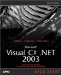When you're working with databases in code, it's important to access data on a cell-by-cell basis. To see how to gain access to the data in a database, we'll create a new example, ch10_03, which will connect to the authors table in the pubs database and display data for various authors in text boxes, as you see in Figure 10.4.

Figure 10.4. The ch10_03 example.To write this example, create a new Windows application named ch10_03 now and connect a SQL data adapter to the authors table. In addition, generate a new dataset, dataSet11 , to hold the entire authors table. Next, add the four multiline text boxes to the example's main form as you see in Figure 10.4, as well as the Get Data button. The text boxes will display data from the first four fields ( au_id , au_lname , au_fname , and phone ) in the authors table.

When the user clicks the Get Data button, we'll start by filling the dataset and displaying the name of each column in the multiline text boxes, as you see in Figure 10.4. To get the name of each column, you access the authors table as dataSet11.Tables["authors"] . This table has a Columns collection, and you can use the ColumnName property of each column to get that column's name:

private void button1_Click(object sender, System.EventArgs e) {  dataSet11.Clear();   sqlDataAdapter1.Fill(dataSet11);   textBox1.Text += dataSet11.Tables["authors"].Columns.ColumnName + "\r\n";   textBox2.Text += dataSet11.Tables["authors"].Columns.ColumnName + "\r\n";   textBox3.Text += dataSet11.Tables["authors"].Columns.ColumnName + "\r\n";   textBox4.Text += dataSet11.Tables["authors"].Columns.ColumnName + "\r\n";   textBox1.Text += "------------" + "\r\n";   textBox2.Text += "------------" + "\r\n";   textBox3.Text += "------------" + "\r\n";   textBox4.Text += "------------" + "\r\n";  .     .     .

Next, we want to get the actual data from the records in the authors table. You access the records in a table with the Rows collection, and you can access an item in a field in a row like this: DataSet11.Tables["authors"].Rows . This returns the value of the second field in the first row in the authors table. Here, then, is how we loop over all the records, showing the data from the first four fields of each record in the application's text boxes:

private void button1_Click(object sender, System.EventArgs e) {   dataSet11.Clear();   sqlDataAdapter1.Fill(dataSet11);     .     .     .  for (int rowLoopIndex = 0; rowLoopIndex <=   dataSet11.Tables["authors"].Rows.Count - 1; rowLoopIndex++) {   textBox1.Text += dataSet11.Tables["authors"].Rows[rowLoopIndex] +   "\r\n";   }   for (int rowLoopIndex = 0; rowLoopIndex <=   dataSet11.Tables["authors"].Rows.Count - 1; rowLoopIndex++) {   textBox2.Text += dataSet11.Tables["authors"].Rows[rowLoopIndex] +   "\r\n";   }   for (int rowLoopIndex = 0; rowLoopIndex <=   dataSet11.Tables["authors"].Rows.Count - 1; rowLoopIndex++) {   textBox3.Text += dataSet11.Tables["authors"].Rows[rowLoopIndex] +   "\r\n";   }   for (int rowLoopIndex = 0; rowLoopIndex <=   dataSet11.Tables["authors"].Rows.Count - 1; rowLoopIndex++) {   textBox4.Text += dataSet11.Tables["authors"].Rows[rowLoopIndex] +   "\r\n";   }  }

That's all it takes to read the data in the various fields in a data table contained in a dataset. You can also write to these fields, accessing them in the same way (don't forget to call the data adapter's Update method to send the changes back to the underlying data source).

Note that you can access the data in the fields of a data row either by numeric index or by name. For example, if you're working with a row of data named row1 , and the au_fname field is the third field in the row, these statements are equivalent:

string firstName = row1["au_fname"]; string firstName = row1;

There's another way to access the data in a dataset if you're only reading datausing data readers, which are fast, low-level data access objects.Microsoft Visual C#.NET 2003 Kick Start
ISBN: 0672325470
EAN: 2147483647
Year: 2002
Pages: 181

Similar book on Amazon